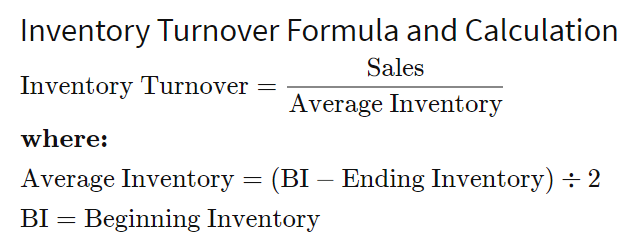# Implication of valuing inventory at NRV

The effects of writedown includes

• Decrease both current and total assets (CA)
• CA = quantity * unit cost, unit cost decreased → CA decreased
• Current ratio (CA/CL) decrease
• CA decreased, CL is the same
• Quick ratio is unaffected
• Quick ratios is based on cash equivalent components, not relating to inventory
• Inventory turnover increase, decrease
• days’ inventory on hand
• Cash conversion cycle
• Decreased total asset → increase total asset turnover → increase debt-to-asset ratio
• Equity decreased → debt-to-equity ratio increased
• Equity = Asset – liability, Liability = constant → equity decrease
• COGS = beginning inventory + purchase – ending inventory
• Value of ending inventory reduced, Purchase and Beginning inventory is fixed
• COGS raised
• Gross margin, operating margin net margin reduced
• Percentage of decrease in net income > percentage of decrease in assets → ROA and ROE are decreased.

Notes:

• Inventory turn-over
•• Total asset turn-onver
•